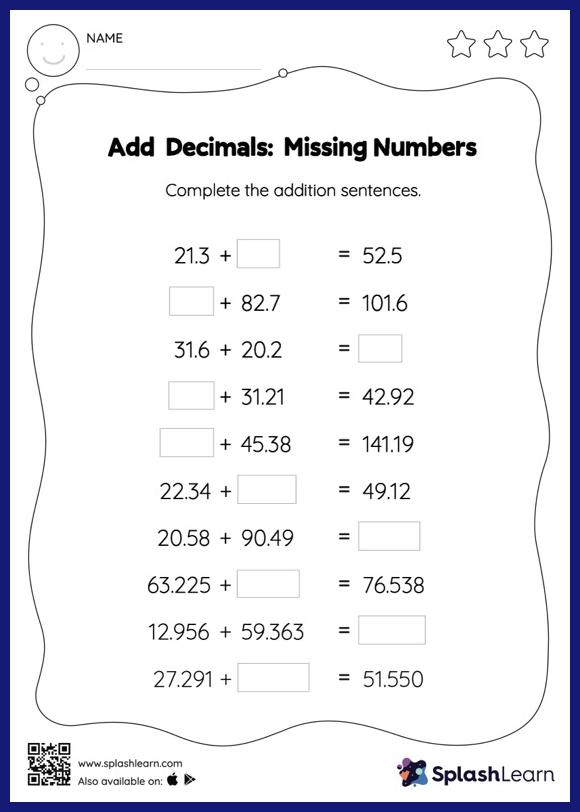# Add Decimals: Missing Numbers Worksheet

Home > Add Decimals: Missing NumbersThis add decimals worksheet has a set of problems curated to ensure that your child becomes more proficient in addition. When adding decimals on this worksheet, students align the decimal points and use zero as a placeholder. Then to find the missing number in the add decimals worksheet, students then apply the relationship between addition and subtraction. In each problem, the numbers are laid out in the horizontal format. Students should try to use different strategies involving composing and decomposing numbers to solve these problems. This will help them develop flexibility and fluency.